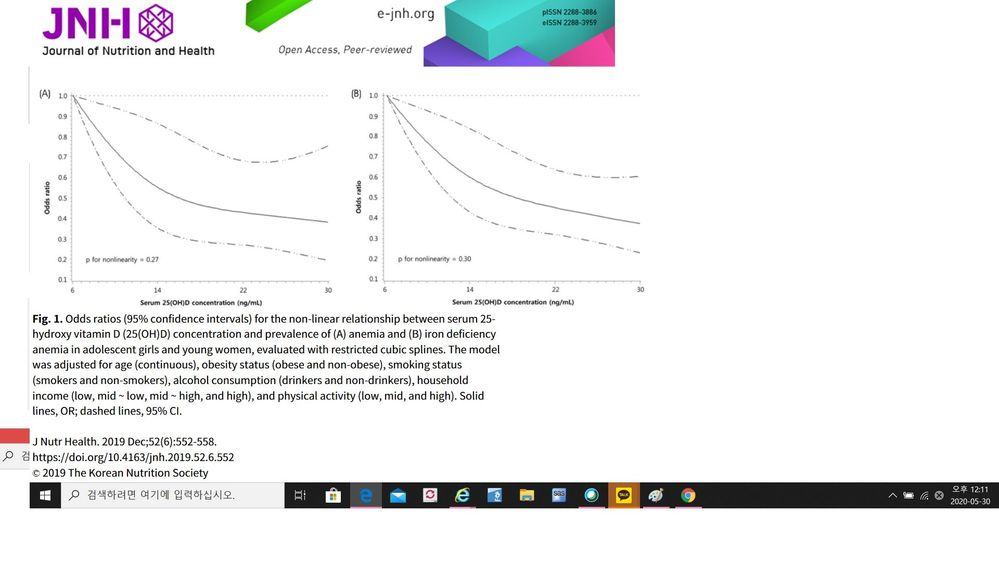## Restricted cubic splines / odds ratio in SAS

Thank you for this very helpful page!
I have used the EFFECT statement together with PROC LOGISTIC. But I haven't figured out how to modify the code in order to plot odds ratios on the y axis, instead of logit or predicted values. Is it possible to do that?

[example]

ods select ANOVA ParameterEstimates SplineKnots;
proc logistic data=cars;
effect spl = spline(weight / details naturalcubic basis=tpf(noint)
knotmethod=percentiles(5) );
model mpg_city = spl / selection=none;
output out=SplineOut predicted=Fit;
quit;

proc sgplot data=SplineOut noautolegend;
scatter x=weight y=mpg_city;
series x=weight y=Fit / lineattrs=(thickness=3 color=red);
run;

[sample figure]